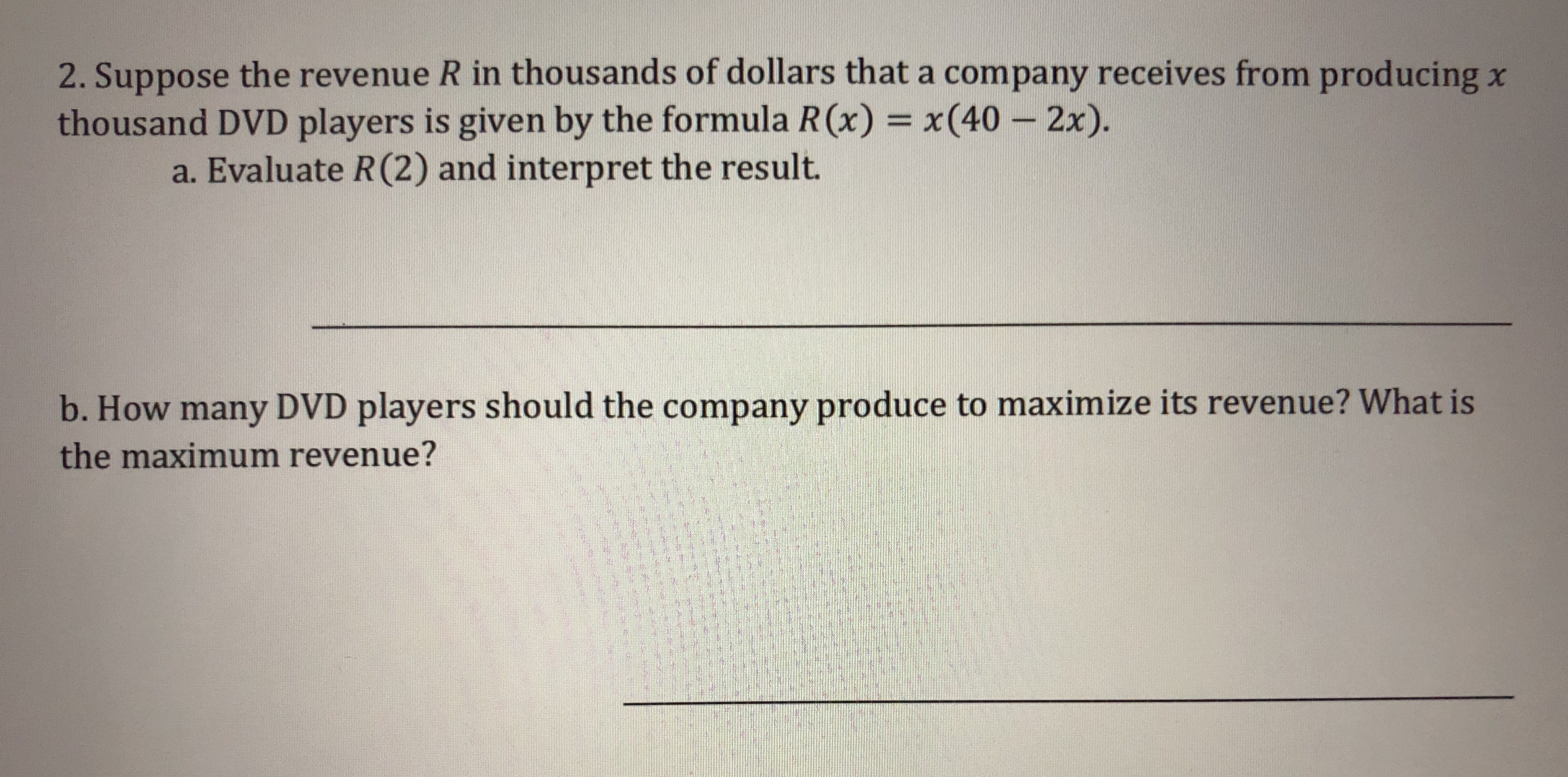# 2. Suppose the revenue R in thousands of dollars that a company receives from producing xthousand DVD players is given by the formula R(x) x(40 -2x).a. Evaluate R(2) and interpret the result.b. How many DVD players should the company produce to maximize its revenue? What isthe maximum revenue?

Question
60 viewshelp_outlineImage Transcriptionclose2. Suppose the revenue R in thousands of dollars that a company receives from producing x thousand DVD players is given by the formula R(x) x(40 -2x). a. Evaluate R(2) and interpret the result. b. How many DVD players should the company produce to maximize its revenue? What is the maximum revenue? fullscreen
check_circle

Step 1

The problem involves the revenue function and its graph (a parabola). Note that the units x and R(x) are in thousands .

Step 2

(Taking into account the units ) R(2) =\$72000 is interpreted as the revenue generated when 2000 units are produced.

Step 3

To determine the maximum revenue , note that the equation R(x)=x(40-2x)=-2x^2+40x represents...

### Want to see the full answer?

See Solution

#### Want to see this answer and more?

Solutions are written by subject experts who are available 24/7. Questions are typically answered within 1 hour.*

See Solution
*Response times may vary by subject and question.
Tagged in

### Equations and In-equations